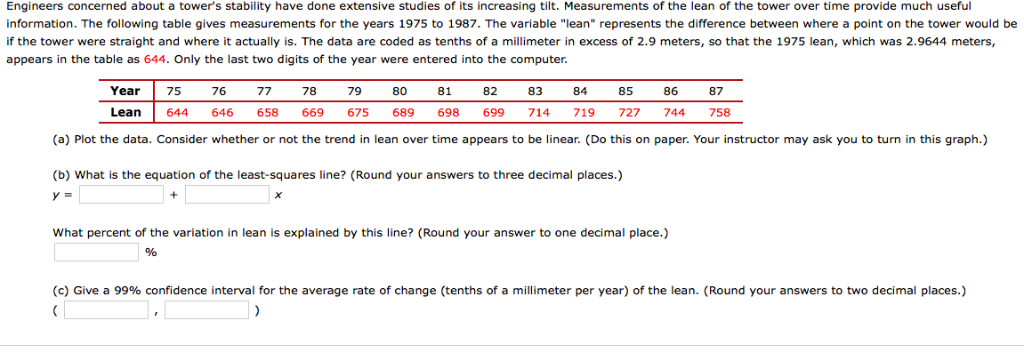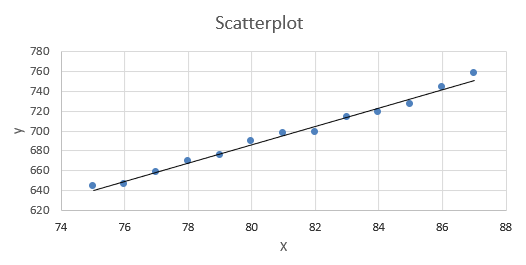# Engineers concerned about a tower's stability have done extensive studies of its increasing tilt. Measurements of...Engineers concerned about a tower's stability have done extensive studies of its increasing tilt. Measurements of the lean of the tower over time provide much useful information. The following table gives measurements for the years 1975 to 1987. The variable "lean" represents the difference between where a point on the tower would be if the tower were straight and where it actually is. The data are coded as tenths of a millimeter in excess of 2.9 meters, so that the 1975 lean, which was 2.9644 meters, appears in the table as 644. Only the last two digits of the year were entered into the computer. Year Lean 75 644 76 646 77 658 78 79 80 81 669675689 698 82 699 83 714 84 719 85 727 86 744 87 758 (a) Plot the data. Consider whether or not the trend in lean over time appears to be linear. (Do this on paper. Your instructor may ask you to turn in this graph.) (b) What is the equation of the least-squares line? (Round your answers to three decimal places.) y = What percent of the variation in lean is explained by this line? (Round your answer to one decimal place.) (c) Give a 99% confidence interval for the average rate of change (tenths of a millimeter per year) of the lean. (Round your answers to two decimal places.)

a)yes, data appears to be linear

b)

 X Y (x-x̅)² (y-ȳ)² (x-x̅)(y-ȳ) 75 644 36.00 2640.38 308.31 76 646 25.00 2438.84 246.92 77 658 16.00 1397.61 149.54 78 669 9.00 696.15 79.15 79 675 4.00 415.53 40.77 80 689 1.00 40.76 6.38 81 698 0.00 6.84 0.00 82 699 1.00 13.07 3.62 83 714 4.00 346.53 37.23 84 719 9.00 557.69 70.85 85 727 16.00 999.53 126.46 86 744 25.00 2363.46 243.08 87 758 36.00 3920.69 375.69
 ΣX ΣY Σ(x-x̅)² Σ(y-ȳ)² Σ(x-x̅)(y-ȳ) total sum 1053 9040 182 15837.1 1688.00 mean 81.00 695.38 SSxx SSyy SSxy

sample size ,   n =   13
here, x̅ = Σx / n=   81.00   ,     ȳ = Σy/n =   695.38

SSxx =    Σ(x-x̅)² =    182.0000
SSxy=   Σ(x-x̅)(y-ȳ) =   1688.0

estimated slope , ß1 = SSxy/SSxx =   1688.0   /   182.000   =   9.2747

intercept,   ß0 = y̅-ß1* x̄ =   -55.8681

so, regression line is   Ŷ =   -55.868 +   9.275 *x

R² =    (Sxy)²/(Sx.Sy) =    0.9885

98.85% of variation is explained by this line

c)

α=   0.05
t critical value=   t α/2 =    2.201   [excel function: =t.inv.2t(α/2,df) ]
estimated std error of slope = Se/√Sxx =    4.06024   /√   182.00   =   0.301

margin of error ,E= t*std error =    2.201   *   0.301   =   0.662
estimated slope , ß^ =    9.2747

lower confidence limit = estimated slope - margin of error =   9.2747   -   0.662   =   8.61
upper confidence limit=estimated slope + margin of error =   9.2747   +   0.662   =   9.94

#### Earn Coin

Coins can be redeemed for fabulous gifts.

Similar Homework Help Questions
• ### Engineers concerned about a tower's stability have done extensive studies of its increasing tilt. Measurements of th...

Engineers concerned about a tower's stability have done extensive studies of its increasing tilt. Measurements of the lean of the tower over time provide much useful information. The following table gives measurements for the years 1975 to 1987. The variable "lean" represents the difference between where a point on the tower would be if the tower were straight and where it actually is. The data are coded as tenths of a millimeter in excess of 2.9 meters, so that the...

• ### Engineers concerned about a tower's stability have done extensive studies of its increasing tilt. Measurements of...

Engineers concerned about a tower's stability have done extensive studies of its increasing tilt. Measurements of the lean of the tower over time provide much useful information. The following table gives measurements for the years 1975 to 1987. The variable "lean" represents the difference between where a point on the tower would be if the tower were straight and where it actually is. The data are coded as tenths of a millimeter in excess of 2.9 meters, so that the...

• ### Engineers concerned about a tower's stability have done extensive studies of its increasing tilt. Measurements of...

Engineers concerned about a tower's stability have done extensive studies of its increasing tilt. Measurements of the lean of the tower over time provide much useful information. The following table gives measurements for the years 1975 to 1987. The variable "lean" represents the difference between where a point on the tower would be if the tower were straight and where it actually is. The data are coded as tenths of a millimeter in excess of 2.9 meters, so that the...

• ### The Leaning Tower of Pisa is an architectural wonder. Engineers concerned about the tower's stability have...

The Leaning Tower of Pisa is an architectural wonder. Engineers concerned about the tower's stability have done extensive studies of its increasing tilt. Measurements of the lean of the tower over time provide much useful information. The following table gives measurements for the years 1975 to 1987. The variable "lean" represents the difference between where a point on the tower would be if the tower were straight and where it actually is. The data are coded as tenths of a...

• ### Correla uneIno sub+ Q Search https://www.webassign.net/web/Student/Assignment-Responses We have enough evidence to conclude that there is a relationship. My Notes Ask Your ngineers concerned about a...Correla uneIno sub+ Q Search https://www.webassign.net/web/Student/Assignment-Responses We have enough evidence to conclude that there is a relationship. My Notes Ask Your ngineers concerned about a tower's stability have done extenslive studies of its increasing tilt. Measurements of the lean of the tower over time provide nformation. The following table gives measurements for the years1 the difference between where a point on the tower would be 975 to 1987. The variable "lean" represents t actually is. The data are coded as...

Free Homework App